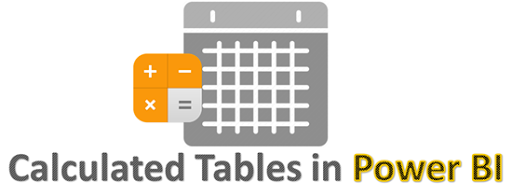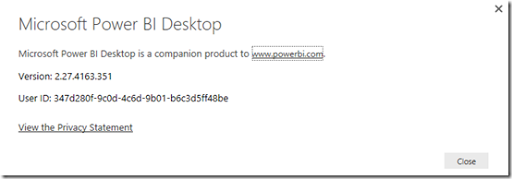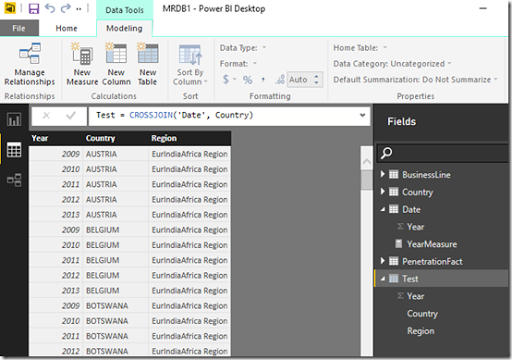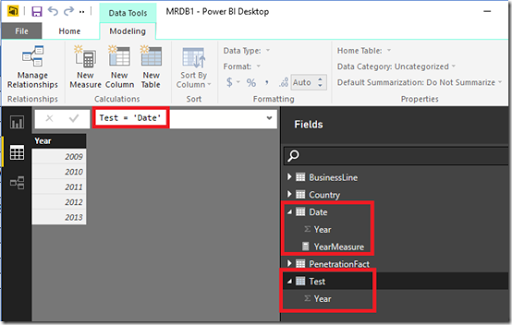# My Thoughts on Calculated Tables in Power BI

Yesterday was a terrific day for all of Microsoft Power BI fans. Microsoft released updates for Power BI Service, Power BI Mobile and Power BI Desktop (with an unbelievable 44 new features) – which basically means no matter whether you are a developer, BI professional or an end user, all of you got something new to play with along with this release. The blogs give a lot of details on what those new features are, so I wouldn’t be going over them. But I wanted to take a moment to pen down a few moments on my thoughts on a new modeling feature within this release – Calculated Tables.Chris Webb has already posted his thoughts on Calculated Tables in Power BI and I am pretty sure Marco Russo / Alberto Ferrari will post some on the same pretty soon (if not, this is an open request from my side for Marco & Alberto to post something on the topic, pretty please) – [Update 9/28/2015 Read Transition Matrix using Calculated Tables]. As usual, a combination of posts from these folks are going to be the best resource for any topic in the Modeling side, and I learn most of my stuff from them. So you would be thinking – what exactly am I trying to achieve in this post? Well, I would like to add my 2 cents on the same and try to see if the community in general agrees with what I think and if not, to learn from the comments and the interaction this might generate.

I) How to build Calculated Tables in Power BI

Before I start on my thoughts on calculated tables, it might be a good idea to quickly see how we can create calculated tables.

1) First make sure that the version of Power BI is equal to or higher than 2.27.4163.351. (I am making a fair assumption that this feature will be enabled in all higher versions also released in the future). If not, download it from here2) Now open any of your existing models in Power BI (or get some new data), and after that, click on the Data tab. You should be able to see the New Table icon in the Modeling tab on the top.3) Click on the New Table icon, and then enter any DAX expression in the format that returns a table TableName = DAX Expression that returns a table Once you do that, then you should be able to see the resultant columns in the new table.II) When is the data in a Calculated Table processed

The official blog says quite a few things on the same-

• A Calculated Table is like a Calculated Column.
• It is calculated from other tables and columns already in the model and takes up space in the model just like a calculated column.
• Calculated Tables are re-calculated when the model is re-processed.

So based on this information, I am going to go a step further and assume that the data in a calculated table is processed during the ProcessRecalc phase of processing. Also, this means that every time any of the source tables changes (like a new calculated column or new data), the data in the calculated table will also change. To prove this, let us try a simple experiment-

1) Make a calculated table called Test which will be the same as the Date table (which currently has just the Year column).Note that measures from the source table are not brought along to the calculated table, which is as expected.

2) Now go to the Date table (which is our source table in this case) and then add a new calculated column called TestColumn with 1 as the value.Note that when we added a calculated column in the source table, the column was replicated in the calculated Table also with the same name. The only difference is that the source table shows an icon for calculated column. This shows that the ProcessRecalc that happens in the source table when a new calculated column is made, also recalculates the calculated table.

III) My thoughts on Calculated Tables

Based on my understanding so far, there are times when I think I should use calculated tables and times when I should not use calculated tables. So here it goes –

a) When NOT to use calculated tables

If you have a way of replicating the calculated table in some form of ETL or source query (even a SQL View), you should not use a Calculated table. Why? A couple of reasons

• If done from ETL / source query, the engine will see the result as a regular table, which means parallel processing of the tables can be done (unlike now, where the ProcessData phase of the source tables have to finish first before the calculated tables can be processed). So calculated tables could lead to slower data processing time.
•  A ProcessRecalc happens every time you do a process full, and adding more calculated tables (as well as calculated columns) unnecessarily will increase the processing time. Also, during development of very large models, you might have to wait for a long time after each calculation is made for the data to appear, since all dependent tables will also have to be recalculated (unless you turn off the automatic calculation mode).
• This one is more an assumption and further research will be needed to validate this, but I am putting it forward anyways. Just like a calculated column is not that optimized for data storage compared to a regular column, I suspect that a calculated table will also not be optimized for storage compared to a regular table. If this is true, this might affect query performance.

b) When to use calculated tables

There are a lot of scenarios where you would want to use calculated tables and I have listed a few of the scenarios below

• During debugging complex DAX expressions, it might be easier for us to store the intermediate results in a calculated table and see whether the expressions ate behaving as expected.
• In a lot of self-service BI scenarios or Prototype scenarios, it is more important to get the results out there faster and hence, it might be difficult or not worth the effort to fiddle with the source queries. Calculated tables can be a quick and easy method to get the desired table structures.
• A kind of scenario that I see very often during prototyping / PoCs is when there are 2 facts in an excel file which are given for making reports. As seasoned users of Power Pivot / Power BI, we know that we will have to create a proper data model with the dimensions. Now, I might need to create a dimension which gets me the unique values from both the tables. For eg, in the below example, I need the Country dimension to get values from both the tables (USA, Canada, Mexico). It might be easier to write an expression for a calculated table like shown below
Country = DISTINCT(UNION(DISTINCT(Table1[Country]), DISTINCT(Table2[Country])))• There are times (like in some role playing dimensions) where you will need to replicate the columns / calculated columns (or even delete) in another table also, even if the columns are built at a later phase in the project. Calculated tables are a perfect match for that, as you will only need to make the changes in one table, and the changes will flow down to the calculated table during ProcessRecalc phase (remember our previous example where we created a TestColumn in the Date table, and the change was reflected in our calculated table also).
• Calculated tables can help in speeding up DAX expressions. This is very similar to the technique of using aggregate tables in SQL database to speed up the calculations in SQL.
• Quick and easy way to create calendar tables (like Chris showed in his blog)

This is still a very early stage as far as Calculated tables are concerned, and I am pretty sure we are going to see some very innovative uses as well as benefits of calculated tables in the days to come. I might also learn that some of my assumptions are wrong, and if I do, I will come back and update this post to the best I can. Meanwhile, feel free to comment on your thoughts / concerns / feedback.

Update – 9/28/2015

Transition Matrix using Calculated Tables – Alberto Ferrari
Use Calculated Table to Figure Out Monthly Subscriber Churn – Jeffrey Wang### Posted by SQLJason

This site uses Akismet to reduce spam. Learn how your comment data is processed.